# Python PIL image library

What is PIL?

This is an old library from 1996.

Support for PIL (Python Imaging Library) got discontinued in 2011, but a project named pillow forked the original PIL project and added support for Python3.

Pillow is now a replacement for PIL.

Pillow supports a large number of image file formats including BMP, PNG, JPEG, and TIFF.

Pillow is frequently used for creating command-line applications that can be used to convert images in various formats.

## PIL.Image.open

The next code shows how to open the image using PIL `Image.open` method:

``````%matplotlib inline
from PIL import Image
from matplotlib.pyplot import imshow
import torchvision.transforms as transforms

pil_img = Image.open(r"apple.jpg")
print(pil_img)

tensor = transforms.ToTensor()(pil_img).unsqueeze_(0)
print(tensor.shape)

pil_image = transforms.ToPILImage()(tensor.squeeze_(0))
print(pil_image.size)
pil_image_again = transforms.ToPILImage()(tensor).convert("RGB")
print(pil_image_again)
print(pil_image_again.size)
display(pil_image_again)
# pil_image_again.show() # not inline
# imshow(pil_img)
``````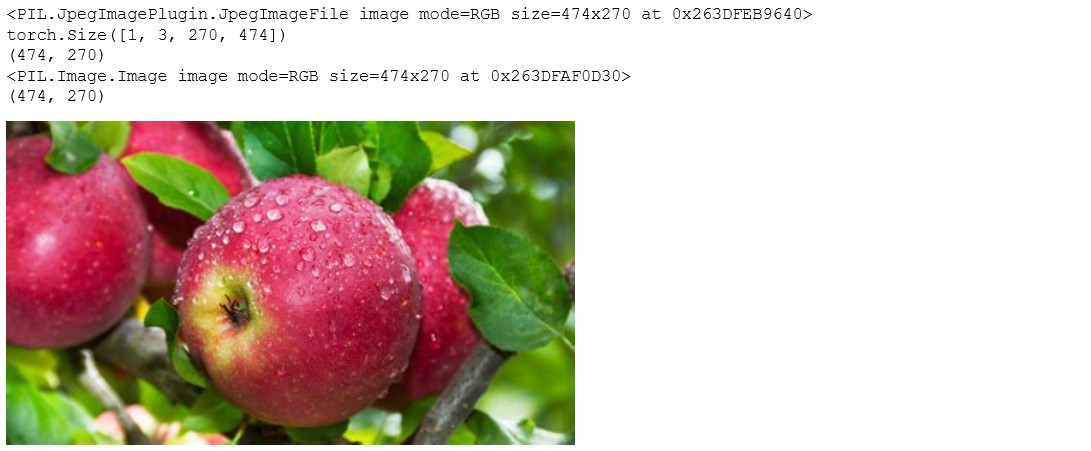The example shows the usage of `PIL.Image.open()` to open a given image file.

`PIL.Image.open()` returns a 2D image.

You can use `torchvision.transforms` to transform the `PIL.Image` to tensor format.

## PIL.Image.mode

RGB images mean they have 3 channels, one for red, one for green and one for blue.

RGBA means there is another alpha channel.

P mode image, that image colors are from the pallette.

A palette with up to 256 different colours is shorter instead of storing 3 bytes for R, G and B for each pixel. P mode image stores 1 byte which is the index into the palette.

The disadvantage of P image mode is that it can only represent 256 unique colours.

L mode image means it is a single channel image. L stands for luminance.

You can check the PIL.Image mode with `mode` method.

``````%matplotlib inline
from PIL import Image
from matplotlib.pyplot import imshow
import torchvision.transforms as transforms

pil_img = Image.open(r"apple.jpg")
print(pil_img.mode)
print(pil_img.convert("L").mode)
print(pil_img.convert("LA").mode)
print(pil_img.convert("P").mode)
print(pil_img.convert("PA").mode)
print(pil_img.convert("RGB").mode)
print(pil_img.convert("RGBA").mode)
``````

Out:

``````RGB
L
LA
P
PA
RGB
RGBA
``````

## Showing the image

We can use `pil_image_again.show()` but it doesn’t work inline from some reason so knowing you will be working inside jupyter notebook as I am aware this method is not the best.

You can simply use the `display()` method that works inline.

Another alternative is to use the `matplotlib.pyplot` method `imshow`.

## PIL functions

The PIL module provides a number of functions we will examine now.

### PIL.ImageFilter

With this we can achieve the EMBOSS effect.

``````from PIL import ImageFilter
img2 = pil_image_again.filter(ImageFilter.EMBOSS)
display(img2)
``````CONTOUR effect as well:

``````img2 = pil_image_again.filter(ImageFilter.CONTOUR)
display(img2)
``````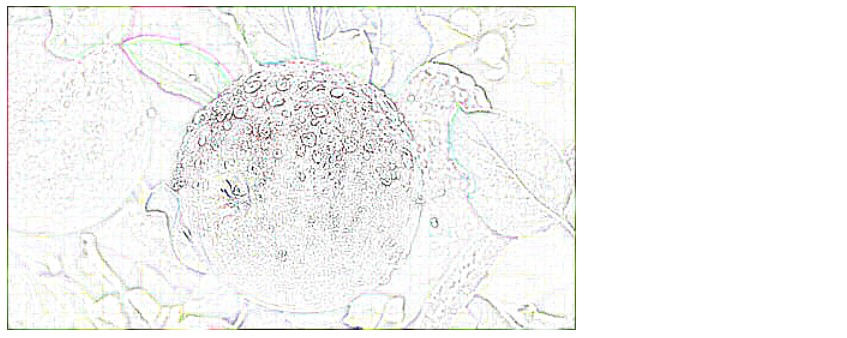MinFilter:

``````img2 = pil_image_again.filter(ImageFilter.MaxFilter(size=5))
display(img2)
``````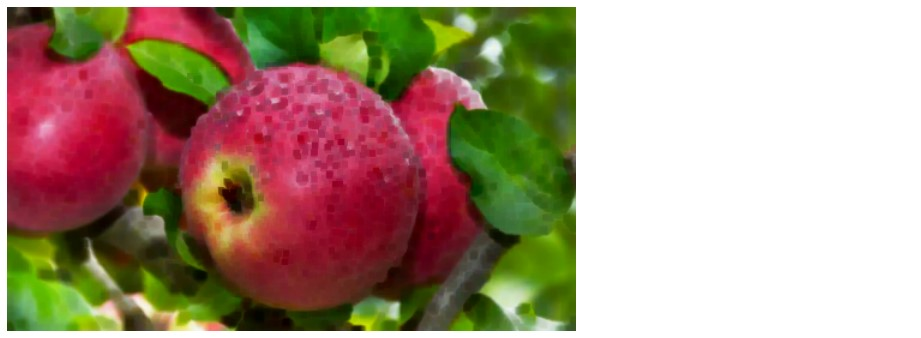MaxFilter:

``````img2 = pil_image_again.filter(ImageFilter.MinFilter(size=5))
display(img2)
``````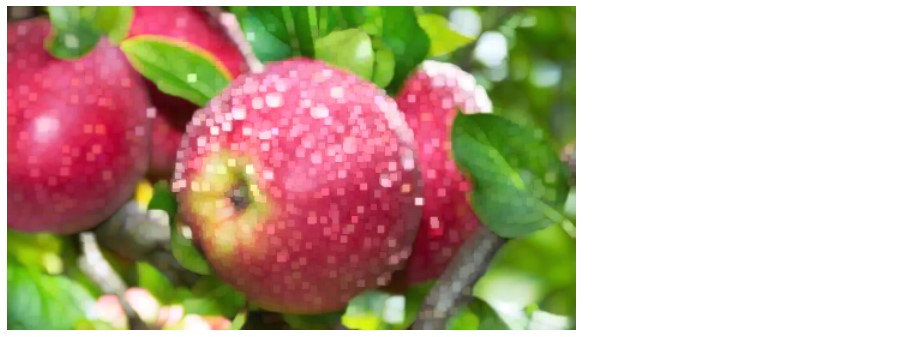If you check

``````help(ImageFilter)
``````

you will find all the possible filters.

### PIL.ImageEnhance

``````from PIL import ImageEnhance
effect = ImageEnhance.Brightness(pil_image_again)
display(effect.enhance(5))
``````Similar to Brightness you can do to Contrast, Sharpness and Color.

### PIL.Image.ImageDraw

`ImageDraw` in PIL (pillow) works like this:

``````import PIL
from PIL import ImageDraw

area = ImageDraw.Draw(pil_image_again)
area.rectangle((0,0, 200, 150), outline="white", width=1)
display(pil_image_again)
``````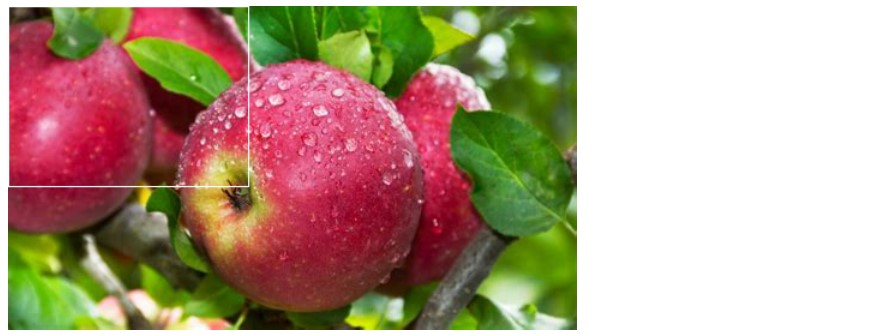You can use `ImageDraw` to draw primitives such as line, point, text, ellipse, polygon, bitmaps, etc.

## Comparison with OpenCV

OpenCV is written in C and C++ whereas PIL is written using Python and C. Because of that OpenCV seems faster.

tags: image & category: python